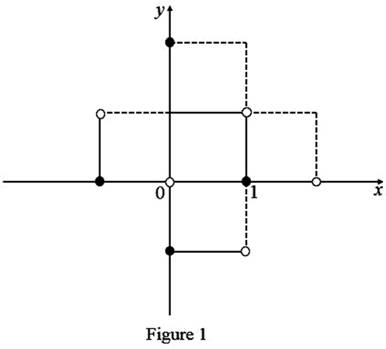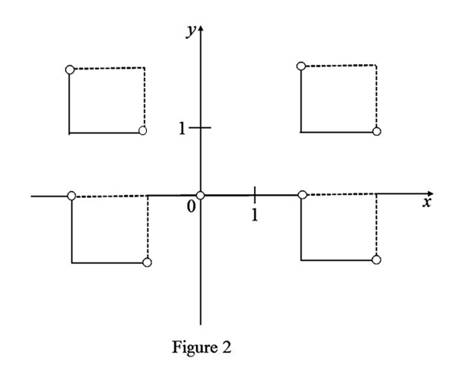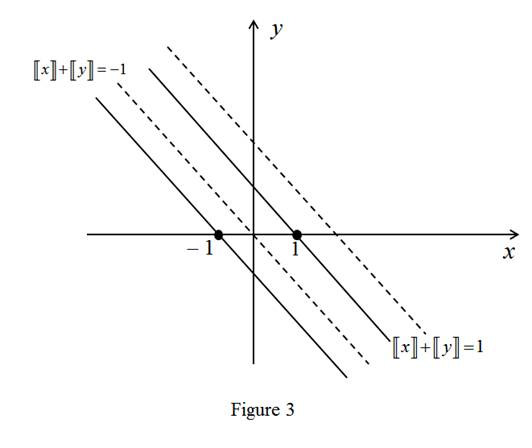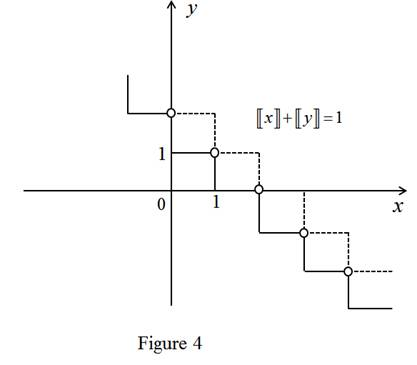# The region in the plane defined by 〚 x 〛 2 + 〚 y 〛 2 = 1 .### Single Variable Calculus: Concepts...

4th Edition
James Stewart
Publisher: Cengage Learning
ISBN: 9781337687805### Single Variable Calculus: Concepts...

4th Edition
James Stewart
Publisher: Cengage Learning
ISBN: 9781337687805

#### Solutions

Chapter 2, Problem 6P

(a)

To determine

## To Sketch: The region in the plane defined by 〚x〛2+〚y〛2=1.

Expert Solution### Explanation of Solution

From the equation x2+y2=1 clearly x2 and y2 are positive integers or zero,

then there are four cases:

(i) If x=1,y=0 then 1x<2 and 0y<1.

(ii) If x=1,y=0 then 1x<0 and 0y<1.

(iii) If x=0,y=1 then 0x<1 and 1y<2.

(iv) If x=0,y=1 then 0x<1 and 1y<0.

(b)

To determine

### To sketch: The region in the plane defined by 〚x〛2−〚y〛2=3.

Expert Solution### Explanation of Solution

Let x=n and y=m then the equation is n2m2=3.

The solutions of the equation n2m2=3 are n=±2 and m=±1.

If n=2,m=1 then (2)2(1)2=41=3

If n=2,m=1 then (2)2(1)2=41=3

If n=2,m=1 then (2)2(1)2=41=3

If n=2,m=1 then (2)2(1)2=41=3

Therefore, {(x,y)|x=±2,y=±1}={(x,y)|2x3  or 2x<11y2  or 1y<0}.

(c)

To determine

### To sketch: The region in the plane defined by 〚x+y〛2=1.

Expert Solution### Explanation of Solution

Given the equation is x+y2=1 then

x+y=1x+y=±1

The region is 1x+y<2 or 1x+y<0.

(d)

To determine

### To sketch: The region in the plane defined by 〚x〛+〚y〛=1.

Expert Solution### Explanation of Solution

Given the equation is x+y=1

For nx<n+1, let x=n, where n is any integer, then

n+y=1y=1n

For any 1ny2n.

### Have a homework question?

Subscribe to bartleby learn! Ask subject matter experts 30 homework questions each month. Plus, you’ll have access to millions of step-by-step textbook answers!